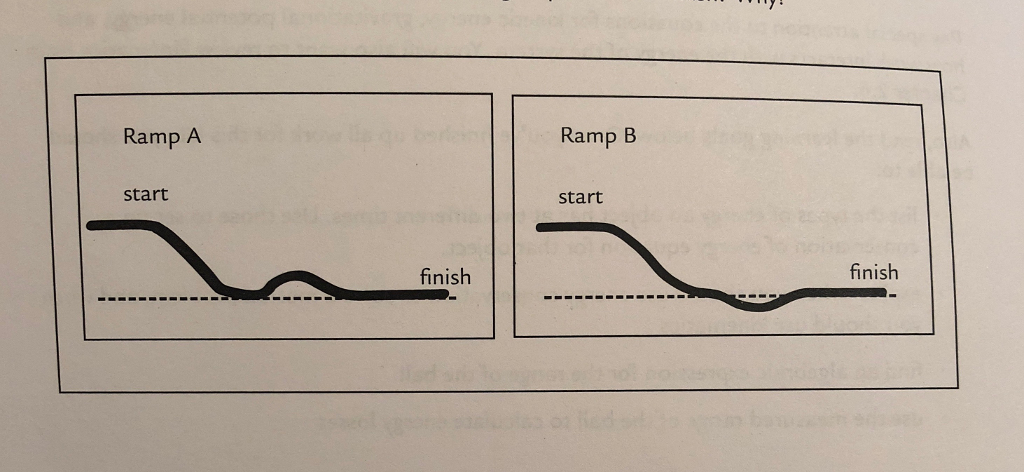# Problem: You release a frictionless cart at the top of each of the 2 ramps. BOTH CARTS ARE RELEASED FROM REST. The ramps have the same height as each other at the starting and finishing points. One has a small bump up then down before the end. The other has a small bump down then up. Which cart has the larger speed at the finish and why?

🤓 Based on our data, we think this question is relevant for Professor Leduc's class at SDSU.

###### FREE Expert Solution

Energy conservation,

$\overline{){\mathbf{m}}{\mathbf{g}}{{\mathbf{h}}}_{{\mathbf{0}}}{\mathbf{+}}\frac{\mathbf{1}}{\mathbf{2}}{\mathbf{m}}{{{\mathbf{v}}}_{{\mathbf{0}}}}^{{\mathbf{2}}}{\mathbf{=}}{\mathbf{m}}{\mathbf{g}}{{\mathbf{h}}}_{{\mathbf{f}}}{\mathbf{+}}\frac{\mathbf{1}}{\mathbf{2}}{\mathbf{m}}{{\mathbf{v}}}_{{\mathbf{2}}}}$

The initial and final heights for the two carts are the same.###### Problem Details

You release a frictionless cart at the top of each of the 2 ramps. BOTH CARTS ARE RELEASED FROM REST. The ramps have the same height as each other at the starting and finishing points. One has a small bump up then down before the end. The other has a small bump down then up. Which cart has the larger speed at the finish and why?